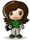# Rounding fun – Banker’s rounding gets me again

posted in: Uncategorized | 0A little suprise you may run into with .NET (particularly if your dealing with monetary amounts) is that the .NET rounding uses roundng-to-nearest (bankers rounding). This rounds off to the nearest even number. For example:

5.15 -> 5.2
5.25 -> 5.2
5.35 -> 5.4
5.45 -> 5.4

Normal arithmetic rounding would result in:

5.15 -> 5.2
5.25 -> 5.3
5.35 -> 5.4
5.45 -> 5.5

Although the .NET bankers rounding is more accurate overall (arithmetic can introduce a slight bias), most people expect arithmetic rounding. The following code performs arithmetic rouding in C#:

Code:

/// <summary>
/// Arithmetic rounding routine for decimals.
/// </summary>
/// <remarks>
/// The normal .NET rounding uses ‘bankers rounding’. When this
/// encounters a tipping point, it rounds to the nearest even number.
/// This rountine implements the more conventional rounding, rounding
/// up on 5 or above, or down otherwise.
/// </remarks>
/// <param name=”d”>The number to round.</param>
/// <param name=”decimals”>Number of decimal places to round to.</param>
/// <returns>The rounded number.</returns>
public static decimal ArithmeticRound (decimal d, int decimals)
{
decimal power = (decimal)Math.Pow(10, decimals);
// Note: converting to positive number before calculating
// rounding, so that we don’t need separate logic for negative
// number handling
decimal result = decimal.Floor((Math.Abs(d) * power) + 0.5m) / power;
return result * Math.Sign(d);
}

For additional information, see: